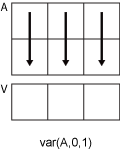# var

## 语法

``V = var(A)``
``V = var(A,w)``
``V = var(A,w,'all')``
``V = var(A,w,dim)``
``V = var(A,w,vecdim)``
``V = var(___,nanflag)``

## 说明

````V = var(A)` 返回 `A` 中沿大小不等于 1 的第一个数组维度的元素的方差。 如果 `A` 是一个观测值向量，则方差为标量。如果 `A` 是一个其各列为随机变量、其各行为观测值的矩阵，则 `V` 是一个包含对应于每列的方差的行向量。如果 `A` 是一个多维数组，则 `var(A)` 会将沿大小不等于 1 的第一个数组维度的值视为向量。此维度的大小将变为 `1`，而所有其他维度的大小保持不变。默认情况下，方差按观测值数量 `-1` 实现归一化。如果 `A` 是标量，则 `var(A)` 返回 `0`。如果 `A` 是一个 `0`×`0` 的空数组，则 `var(A)` 将返回 `NaN`。 ```

``V = var(A,w)` 指定权重方案。如果 `w = 0`（默认值），则 `V` 按观测值数量 `-1` 实现归一化。如果 `w = 1`，则它按观测值数量实现归一化。`w` 也可以是包含非负元素的权重向量。在这种情况下，`w` 的长度必须等于 `var` 将作用于的维度的长度。 `
`当 `w` 为 0 或 1 时，`V = var(A,w,'all')` 计算 `A` 的所有元素的方差。此语法适用于 MATLAB® R2018b 及更高版本。`

``V = var(A,w,dim)` 返回沿维度 `dim` 的方差。要维持默认归一化并指定操作的维度，请在第二个参数中设置 `w = 0`。`

`当 `w` 为 0 或 1 时，`V = var(A,w,vecdim)` 计算向量 `vecdim` 中指定维度的方差。例如，如果 `A` 是矩阵，则 `var(A,0,[1 2])` 计算 `A` 中所有元素的方差，因为矩阵的每个元素包含在由维度 1 和 2 定义的数组切片中。`

``V = var(___,nanflag)` 指定在上述任意语法的计算中包括还是忽略 `NaN` 值。例如，`var(A,'includenan')` 包括 `A` 中的所有 `NaN` 值，而 `var(A,'omitnan')` 则会忽略这些值。`

## 示例

```A = [4 -7 3; 1 4 -2; 10 7 9]; var(A)```
```ans = 1×3 21.0000 54.3333 30.3333 ```

```A(:,:,1) = [1 3; 8 4]; A(:,:,2) = [3 -4; 1 2]; var(A)```
```ans = ans(:,:,1) = 24.5000 0.5000 ans(:,:,2) = 2 18 ```

```A = [5 -4 6; 2 3 9; -1 1 2]; w = [0.5 0.25 0.25]; var(A,w)```
```ans = 1×3 6.1875 9.5000 6.1875 ```

```A = [4 -2 1; 9 5 7]; var(A,0,1)```
```ans = 1×3 12.5000 24.5000 18.0000 ```

`var(A,0,2)`
```ans = 2×1 9 4 ```

```A(:,:,1) = [2 4; -2 1]; A(:,:,2) = [9 13; -5 7]; A(:,:,3) = [4 4; 8 -3]; V = var(A,0,[1 2])```
```V = V(:,:,1) = 6.2500 V(:,:,2) = 60 V(:,:,3) = 20.9167 ```

```A = [1.77 -0.005 3.98 -2.95 NaN 0.34 NaN 0.19]; V = var(A,'omitnan')```
```V = 5.1970 ```

## 输入参数

• `0` - 按观测值数量 `-1` 实现归一化。如果只有一个观测值，则权重为 1。

• `1` - 按观测值数量实现归一化。

• 由非负标量权重构成的向量，这些权重对应于沿其计算方差的 `A` 的维度。

• 如果 `dim = 1`，则 `var(A,0,1)` 返回包含每一列中元素的方差的行向量。• 如果 `dim = 2`，则 `var(A,0,2)` 返回包含每一行中元素的方差的列向量。`dim` 大于 `ndims(A)` 时，`var` 返回一个大小与 `A` 相同的由零值组成的数组。`NaN` 条件，指定为下列值之一：

• `'includenan'` - 包含 `NaN` 值的输入的方差也为 `NaN`

• `'omitnan'` - 出现在输入数组或权重向量中的所有 `NaN` 值都将被忽略。

## 详细信息

### 方差

`$V=\frac{1}{N-1}\sum _{i=1}^{N}|{A}_{i}-\mu {|}^{2}$`

，其中，μ 是 A 的均值，

`$\mu =\frac{1}{N}\sum _{i=1}^{N}{A}_{i}.$`